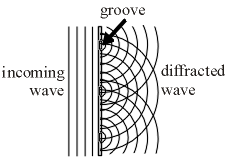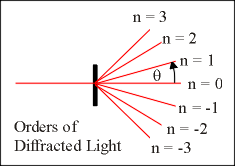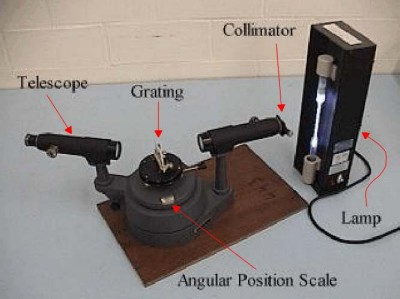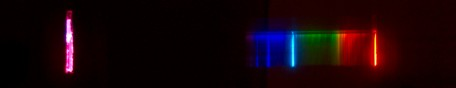### A diffraction grating shows the distinct line pattern of a plasma.

WATCH VIDEO

Teachable Topics:

• Emission spectrum
• Diffraction

Theory:

All elements emit light energy at specific wavelengths when ionized by an electric current. A spectroscope separates the light by sending each wavelength into a slightly different direction. The resulting spectrum appears as a series of bright emission lines (the photo above shows some of these lines). The pattern of these lines is very distinct to each atom, and just as police can use a person’s fingerprints to identify them, physicists can use a gases' spectrum to identify which elements it contains.

The fact that atoms give off only certain wavelengths says something important about electrons in atoms. A physicist named Max Planck theorized that light came in bits called “photons”. He predicted (correctly!) that each photon would have an energy E related to its wavelength l by this equation:

E = hc/l

in which c is the speed of light and h = 6.626176x10-34 Js is called “Plank’s constant”. For an atom to give off a photon of light, an electron in that atom has to lose some of its energy. If an atom’s electron could lose energy in any arbitrary amount, then we would expect that an atom could emit any wavelength (and thus, any colour). However, we observe only discrete colours, which implies that atoms can have only certain discrete energies.

To be able to see the discrete colours given off by a gas, you first have to be able to break the light up into its component colours. One way to do this is with a diffraction grating, which is a piece of clear plastic with thousands of microscopic grooves all etched along a certain direction. When light passes through the grating, it spreads out in a “ripple” pattern from each groove as shown in Figure 1.Figure 1: Diffraction grating

As all waves do, the light waves interfere with one another to reinforce each other in some directions and to cancel one another out in other directions. A beam of light of a single colour (monochromatic light) with wavelength l passing through a grating will be deflected by an angle l. This angle l is the angle between the deflected beam of light and the beam that passes straight through. The amount of deflection depends on the spacing of the grooves in the grating, d. All these variables are related through the following equation: nl = d sin(lThe variable n is called the order number. Since the incoming light of wavelength l gets split into several beams, n provides a label to tell one beam of light from the others.Figure 2: Diffraction

Apparatus:

• Spectroscope
• Lamp and gas tube
• Diffraction grating

Procedure:

• Assemble the spectroscope, and rotate the telescope until it lines up with the collimator on the other side of the spectroscope.
• Flick the switch on the lamp to turn the power on. Line the tube up with the collimator so that when you look through the telescope, you see a bright, clear line that is the colour of the light from the tube (in the photo above, this is the bright pink line to the left).
• Rotate the telescope to the right to see the spectal lines. Now rotate the the telescope so you can see the lines to the left of the central one. They should come in the same order as the first set of lines. These lines are the first-order (n=1) spectral lines. If you rotate farther to the right or left, you’ll find the pattern repeats itself over again. These are the second, third, etc. order lines.Figure 3: Apparatus

Results for Hydrogen: